Search Over 1 Million OEM Parts
Most parts in stock- Same day fulfillment

# Basic Electrical Theory

updated

## The Fundemental Laws of Electricity

Electron- Negative charge
Proton- Positive charge

Measuring Electricity :

Electrical Power - Watts ; Which is equal to ¨Volts times ¨Amps
Electrical Quantity - Amps
Electrical Pressure - Volts
Electrical Resistance - Ohms
I = Current (amps)

Insulators - Substances that WON´T carry electricity
Conductors - Substances that allow for electricity to flow

Electricity is a force of nature, a form of energy, like heat or gravity. In most of nature, electrons and protons are present in equal numbers, and their negative and positive charges cancel each other out. That's why you can't tell there are any charges there. Everything from the Rocky Mountains to your eyelashes contain incredible number of electrons.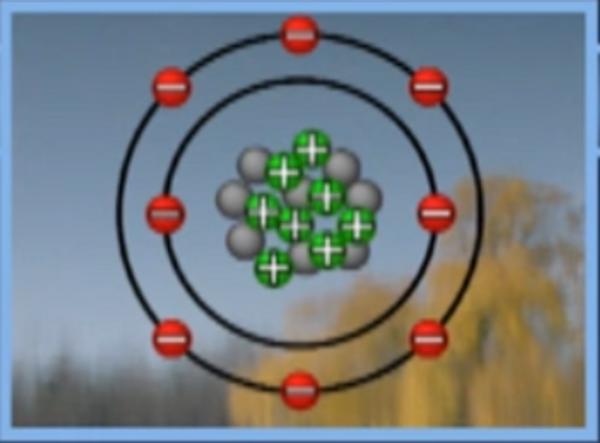Atom

Electricity is best described as a flow of electrons through a medium usually a conducting material. Electrons are subatomic particles that orbit the nucleus of most atoms and the manipulation of these electrons is how you get an electrical current.

Each electron has a tiny charge, a force that draws it toward the protons locked in the atom's nucleus.

The phenomenon where opposite electrical charges attract and like charges repel each is a fundamental law in nature.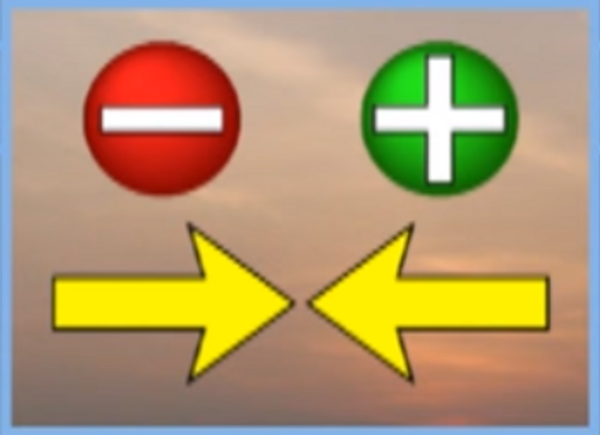Electrons have a tiny charge that draws it towards protons locked in the atom´s nucleus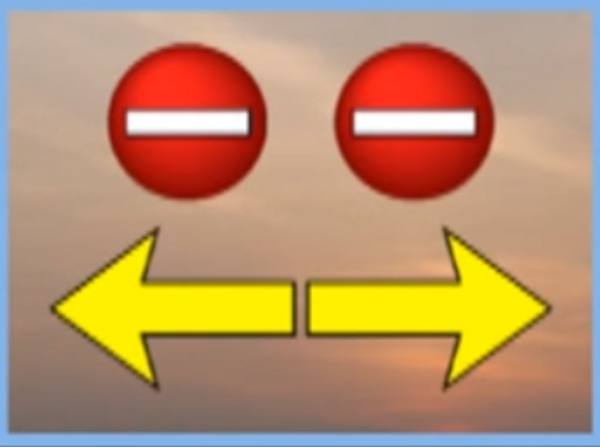The electron´s negative charge tries to push it away from other electrons

When a suitable path connects two potentially opposite charged terminals they pass electrons creating an electrical current.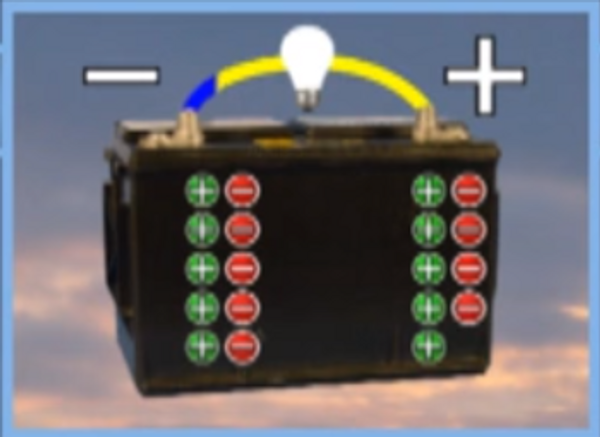For example on a battery, there are excess electrons in the negative terminal, those electrons repel each other driving some of them away from the terminal and at the same time, the positive charge at the other terminal attracts electrons.

A good analogy is to think of electricity as water flowing under pressure. For example, water sitting by itself cannot do any work, but if you pressurize it, by using gravity or a pump, you can make it perform work.

An important concept to remember is that, for our purposes, electricity can only travel in a complete circuit.Electricity passing through a complete circuit.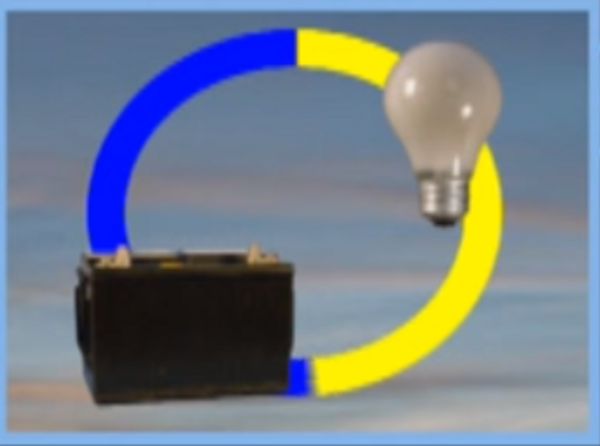In its simplest form, an electrical circuit generally includes a source, such as a battery or generator, and a load such as light bulb or electrical motor.

By using conductors and insulators, we can easily control electricity´s path and make it do what we want. Electricity travels relatively easily through any metal. It travels through water, if certain compounds, such as salt or sugar, are dissolved in that water. Also, electricity will not flow in significant amounts through most other substances - including glass, plastic, and air - unless the energy level is exceptionally high -- like lightning.

## Measuring Pressure :

Like a water wheel, the amount of work we can get an electrical circuit to perform depends on several factors. One factor is the pressure of the water, if we increase the pressure, we can make the water wheel turn faster, and make it do more work.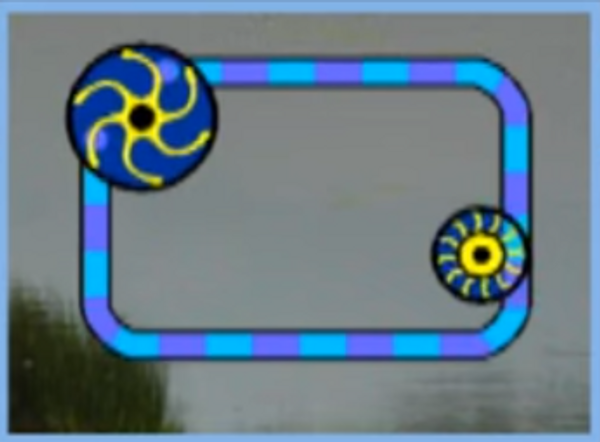Water Wheel Analogy

In electricity, this pressure is called voltage, and it's measured in units called volts. The more voltage, or pressure, that a source has, the more work you can get it to perform. For example, increasing voltage will make a motor run faster or a light bulb burn brighter.

Another factor is the amount of water flowing. If we install a T configuration on our circuit and add another water wheel, it will require more water to operate the two wheels.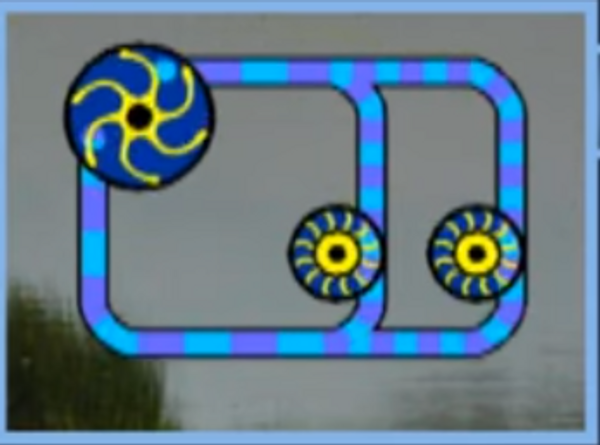T-Configuration Circuit

In electricity, this amount of water is called current or amperage. The way we quantitatively measure units of electricity is by expressing the units in amperes, or amps for short. In this way, we say that a given circuit draws a certain number of amps.

Like in this water circuit we have created if we increase the pressure of the pump or use bigger pipes allowing more water to flow so too can an electrical system increase its flow of electricity. By increasing its voltage or reducing the resistance of a circuit this will cause the amperage to increase.

If you want to measure how much power is behind our water circuit, you would measure how much water is flowing through it, and the pressure of that water.
Likewise, the amount of electrical power flowing through a circuit depends on both the amperage and the voltage.

Electricity is measure in watts which is Volts X Amps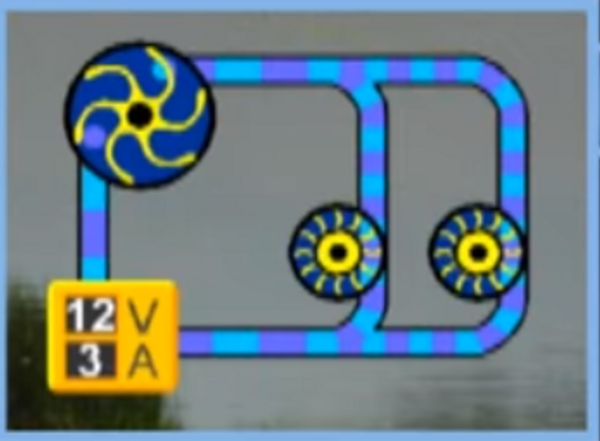For example a 12 volt circuit that draws 3 amps is rated as being 36 watts

The water wheel slows the water flowing in it, reducing the amount of water we can push through it. The pipes connecting the pump to the water wheel restrict the water flow further.
In electricity, we call this resistance and it is measured in units called Ohms. All circuits have some resistance in them hence we must supply enough volts and amps to overcome this resistance to make the circuit work properly.

The voltage, amperage and resistance of a circuit are related to each other through a series of equations called Ohm´s Law. I=V/R

## Electrical Circuits:

1. Series - All current flows through all components

2. Parallel Circuits - Components are connected side-by-side so the current flow is divided among the components.

Let us assume that our components in an electrical circuit have the same resistance when you connect them in series. The amperage flowing through both is the same; but the voltage acting on each component is cut in half.

For example, if we connect two motors in series and supply them with 12 volts and 6 amps, each motor will receive 6 amps -- but only 6 volts. By the same token, if you added a third motor, each motor would still receive 6 amps -- but only 4 volts.
If we connect two motors in parallel, each motor will receive the full 12 volts -- but only 3 amps.

These principles also hold true for electrical sources. For example, our 12-volt battery is actually six cells that generate 2 volts each. Connected in series, they give us our 12 volts.

### Cautions :

1. If the motors had different resistance, distribution of volts or amps would not be equal, since electricity always seeks the path of least resistance.

2. Not all circuits are easily classified as series or parallel. Many circuits contain a number of components and may have some of them connected in series and others in parallel.

## Recap

Electricity is a force of nature – a form of energy – like light, heat, or gravity. Electricity and
magnetism are closely related. They are two forms of the force we call electromagnetism.

• Electrical current is the flow of electrons.
• Voltage is the force, or pressure, that causes the electrons to flow in a circuit.
• Ampere is the unit of measure used to describe the amount of electrons
flowing in a circuit.
• Ohm is the unit of measure used to describe resistance to electron flow.
Conductors and insulators control the path of electricity in a circuit.
• Conductors carry the electricity in a circuit.
• Insulators restrict the flow of electricity in a circuit.
Electricity can only travel in a complete circuit (unless the voltage is extremely high). There are
two basic kinds of electrical circuits: series and parallel.
• In a series circuit, the electrical components are connected so all the current flows
through all the components.
• In a parallel circuit, the components are connected side by side so the current flow is
divided among the components.